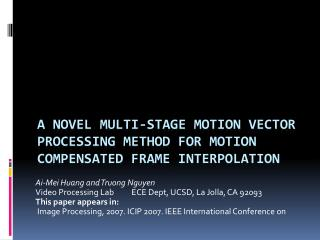DownloadDownload PresentationA NOVEL MULTI-STAGE MOTION VECTOR PROCESSING METHOD FOR MOTION COMPENSATED FRAME INTERPOLATION

# A NOVEL MULTI-STAGE MOTION VECTOR PROCESSING METHOD FOR MOTION COMPENSATED FRAME INTERPOLATION

Télécharger la présentation## A NOVEL MULTI-STAGE MOTION VECTOR PROCESSING METHOD FOR MOTION COMPENSATED FRAME INTERPOLATION

- - - - - - - - - - - - - - - - - - - - - - - - - - - E N D - - - - - - - - - - - - - - - - - - - - - - - - - - -
##### Presentation Transcript

1. Ai-Mei Huang and Truong Nguyen Video Processing Lab ECE Dept, UCSD, La Jolla, CA 92093 This paper appears in: Image Processing, 2007. ICIP 2007. IEEE International Conference on A NOVEL MULTI-STAGE MOTION VECTOR PROCESSING METHOD FOR MOTIONCOMPENSATED FRAME INTERPOLATION

2. Overview • Introduction • Block diagram of the proposed algorithms • Prediction residual energy analysis • The proposed multi-stage motion vector processing method • Simulation results • Conclusions

3. Introduction • Motion-compensated frame interpolation (MCFI) improves temporal quality by increasing the frame rate at the decoder. • Frame interpolation for compressed video remains a problem due to the use of improper MVsare often generated. • The proposed algorithms preserve the object structure informationbut also produce a smoother motion vector field (MVF).

4. Block diagram of the proposed algorithms

5. Prediction residual energy analysis(1/5) • In , we have discussed that there exists a strong correlation between MV reliability and its associated residual energy. • These high residual energies regions are distributed over where object edges are located. • Let vm,n denote the MV of each 8×8 block. We classify vm,ninto three different reliability levels, reliable, possibly reliable.

6. Prediction residual energy analysis(2/5) • For a MB (16×16) with only one MV, we simply assign the same MV to all four 8×8 blocks(bm,n): • If Em,n≧ ε1 , it will be considered as unreliable(L1). • Consider intra-coded MBs as unreliable(L1). • The neighboring of L1MBs or MVs in the same MB will be classified as possibly reliable (L2). • Other MBs will be classified as reliable (L3).

7. Prediction residual energy analysis(3/5) Motion Vector Reliability Map

8. Prediction residual energy analysis(4/5) • Analyze the connectivityof the unreliable MVs in MVRM and create a MB merging map. • If a MB that has unreliable MVs connecting to other unreliableMVs in vertical, horizontal or diagonal directionsin adjacent MBs, these MBs will be merged. • The merging process is performed on a MB basis using MVRM, and all MBs will be examined in a raster scan order. • The 32×32 block size is the maximumfor merging.

9. Prediction residual energy analysis(5/5) • The diagonal direction is not considered for intra-intra MB merging, because the possibility for two diagonal intra-coded MBs belonging to the same object is lower. (c) MV reliability classification map. Unreliable and reliable MVs are marked in yellow and white colors, respectively. Intra-coded MBs are marked in cyan color. (d) MB merging map.

10. Block diagram of the proposed algorithms

11. The proposed multi-stage motion vector processing method(1/4) • Find the best MV for each merged group: • If the ABPD of v*bis less than a threshold ε2 ,assignv*bto the merged MBs in Cu. • Otherwise, drop the selected MV(v*b) and wait until a proper MV propagates to its neighborhood in next iteration. • Process stops until all merged groups have been assigned new MVs. S denotes the reliable MVs in merge group & adjacent blocks. Cu denotes the merged group.

12. The proposed multi-stage motion vector processing method(2/4) • Reclassify MV reliability based on BPD resulted from the selected MV. • BPD(m, n) of each 8×8 block is obtained by simply summing up difference error like Eq(1). • If BPD(m, n) is higher thanε3,vm,n is unreliable(L1). • Otherwise, other MVs will be classified as reliable(L2). • If the MB consists of multiple motion, those unreliable MV can be easily detected by BPD.

13. Block diagram of the proposed algorithms

14. The proposed multi-stage motion vector processing method(3/4) • For those unreliable MVs of 8×8 blocksin the updated MVRM, correct them by using a reliability and similarity constrained vector median filter: Scontains the neighboring MVs centered at vm,n di,jdenotes the distance between vi,j and vm,n Vm,n

15. The proposed multi-stage motion vector processing method(3/4) • For those unreliable MVs of 8×8 blocks in the updated MVRM, correct them by using a reliability and similarity constrained vector median filter: • Two MVs are considered to be similar if the angle distance(di,j) is below a threshold, ε4. • Before updating v*m,nin MVF2, check on BPD of v∗m,n to ensure that error energy is descended. • If the energy check fail, correct it in next iteration. Scontains the neighboring MVs centered at vm,n di,jdenotes the distance between vi,j and vm,n

16. Block diagram of the proposed algorithms

17. The proposed multi-stage motion vector processing method(4/4) • MV smoothing process in  to reduce visual artifacts due to high BPD. • On the frame boundary, using unidirectional interpolation based on the directions of MVs:

18. Simulation results(1/3)

19. Simulation results(2/3)

20. Simulation results(2/3)

21. CONCLUSIONS • We propose a novel algorithm based on the received information for MCFI . • Accomplishing the concept of object motion without complex motion estimation. • The method outperforms other conventional methods on both objective and subjective video quality.